Solar Envelope  A construction method using AutoCAD 2000 by Thanos N. Stasinopoulos A solar envelope is an imaginary surface covering a site like a roof based on the perimeter of the plot. The slope of its facets is adjusted so to avoid shading of the surroundings during a certain period. The form of a solar envelope depends on the plot (=contour shape & ground slope) and on the lowest position of the sun during the period when insolation has to be available. This is usually taken on the Winter Solstice (December 21st), at moments symmetrical to solar noon (e.g. 9:00 & 15:00). Solar envelopes are employed as a planning restriction regulating the maximum building height on a site in order not to obstruct solar access onto adjacent land ('solar rights'). The building density which results in this manner can be increased in two ways, by elevating the base of the solar envelope and by reducing the minimum duration of insolation. The geometric construction of a solar envelope is rather complicated, especially for a complex contour or sloping ground. The process is quite easier by using a solid modeler like AutoCAD 2000, as follows:

 Step 1: Data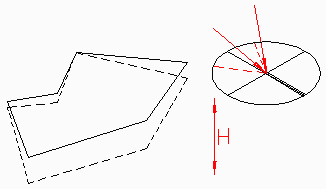The example illustrated here refers to a plot with a concave perimeter on sloping ground, where the maximum permitted building height is H. Having established the two end moments of insolation, each direction of solar rays is set according to solar azimuth & altitude for the given latitude, day of the year and hour. That data can be obtained through the Distant Light / Sun Angle Calculator section of AutoCAD or from a sun path diagram. [The dotted lines show the horizontal projections of the plot and the solar rays at the selected moments.] Step 2: Height solid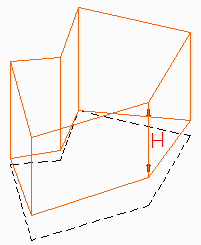A vertical Line of length H is drawn from the topmost vertex of the perimeter. That line is used as Path in order to Extrude the perimeter into a solid volume. Step 3: Cropping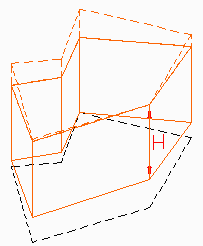The top of the solid is not parallel to the ground but horizontal. The extra part is cut off by Copying the solid vertically at distance H, then Subtracting the copy from the original. The final solid defines the maximum height H at any point of the plot. Step 4: Solar direction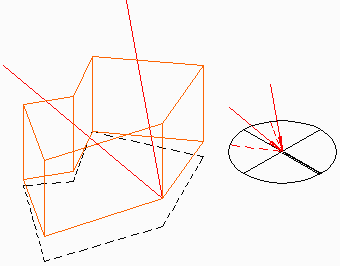Two additional Lines are drawn from the same topmost vertex as in Step 2, parallel to the set solar directions. The top ends of these lines should be substantially higher than the solid volume. Step 5: Solar volumes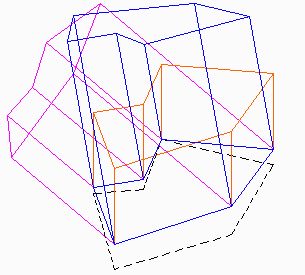The two lines from Step 4 are used as Paths in order to Extrude the perimeter into two new solids. All points within these two solid volumes cast shadows inside the plot during the two end moments. Step 6: Solar envelope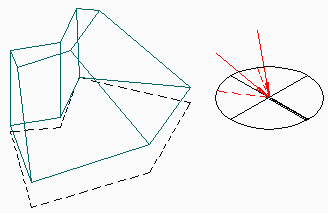The solar envelope is the Intersection of the three solids constructed in Steps 3 & 5. The shadows of all points within the solar envelope move inside the plot during the selected time period. This is due to the fact that, during that time, the sun moves at higher altitude than on the end moments, therefore shadows approach the southern side. Furthermore, the sun is closer to North-South axis, bringing shadows towards the middle of the plot. Remarks The same method is applied if the solar envelope base is set higher from the ground in order to increase building density. If the vertices of the perimeter are not co-planar, then the plot is divided into co-planar sections. Steps 2-5 are followed on each section as for separate plots, then the intersection of Step 6 is applied to all solids.
 CommentsTNS main pagePage posted 18.3.00; last edited 09.07.01